# fractal Dimension

You hear the term dimension very often in sci-fi movies. The fictional space-ships that move at warp speeds & hastily disappear into hyperspace (space with higher dimensions).

From our knowledge of elementary geometry, we know that a line has dimension 1, a square has dimension 2, a cube has dimension 3, and so on. Have you noticed that when we hear about dimensions, we always hear about them in terms of whole numbers (1,2,3,4, etc.)?

What if someone tells you that there are objects of "fractal" dimension, like 1.5 or 2.6? You would be surprised, wouldn't you? So was I !

Before we see what these objects are and what makes these objects have a fractal dimension, let's understand how a dimension is defined. We will make use of a property called self similarity, to invent our new definition of dimension. By self similar, we mean that a small part of the object, when magnified by a certain extent, appears same as the original object.

Consider the line segment below. It can be separated into 4 = 4^1 "miniature" pieces. Each is 1/4 the size of the original. Each looks exactly like the original figure when magnified by a factor of 4. Let's call this "magnification factor" as scaling factor. In this case, the scaling factor is 4.Similar to the line segment above, a square can be separated into miniature pieces with each side = 1/4 the size of the original square. However, we need 16 = 4^2 pieces to make up the original square figure. Also, each piece , when magnified by a factor of 4, will appear same as the original square.Continuing the analogy, a cube can be separated into 64 = 4^3 pieces with each edge 1/4 the size of the original cube.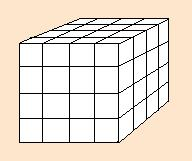Based on the 3 examples above, we can tabulate our findings as: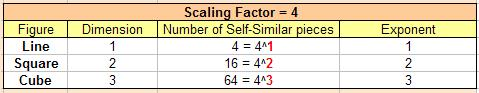We notice that the dimension of the figure, always matches the exponent of the scaling factor. Is it a co-incidence?
Lets try with some other scaling factor, say 3. Here, we divide a line into 3 equal parts, and so on.Once again, we find that the dimension of the object matches the exponent of the scaling factor. Co-incidence? No!
Based on our observations above, we arrive at a new definition of dimension.

The number of selfsimilar pieces in the final figure (N) = (scaling factor) ^ Dimension
or simply, N = S ^ D

This formula for dimension was first defined by Hausdorff and is called as Hausdorff dimension. The dimension we come across in general terms or in every day life is called as Topological dimension. A topological dimension is always a whole number (hence we never heard of a fractal topological dimension before!).

How about Hausdorff dimension, can it be a fraction? Lets see...
Using our formula, N = S ^ D.
Taking Log (base 10) on both sides
Log(N) = D x Log(S) , which implies

D = Log(N) / Log(S) ---------------------- (1)

You may notice that since D is of the form a upon b, it can be a fraction! In other words, we can say that, the way Hausdorff dimension is defined for self similar objects, the dimension can be a fraction.

Now comes more interesting part. We will create a figure, that has fractal (Hausdorff) dimension.
Lets start with a line of length 1. The length does not matter here.Lets divide the line into 3 equal parts, and chop it off in the middle. What we get is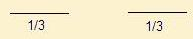What's the scaling factor here? Remember, Scaling Factor = Magnification Factor? So, if we magnify each of the 1/3 rd part 3 times, then we get back the original line segment. Hence scaling factor = 3.
Now, lets join the 2 broken parts again, but with a inverted V bridge, each side of the V being equal to 1/3rd of the original line segment.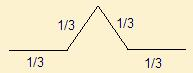(Fig. I)
We repeat the same process with each of the 1/3 rd segments. We getAfter several such repetitions, we obtain a figure like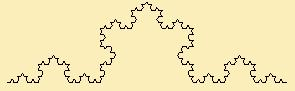This figure is also known as Koch curve. What is the dimension of this figure? We will calculate this in a moment. But thinking intuitively, this figure is not exactly a curve. It is also not a one dimensional figure (eg. a line). So its dimension must be somewhere between 1 & 2.

Lets find out! We will use the new definition of dimension we just invented.
D = Log(Number of self similar pieces) / Log(scaling factor)
From figure 1, we already know the scaling factor, which is 3. How many self similar pieces are there in the figure? We have 4 of them (2 on the side and 2 that make up the inverted-V bridge)

So, D = Log(4) / Log (3), which turns out to be 1.26. The dimension of Koch Curve is 1.26. We just "invented" an object with fractal dimension! Though the idea of fractal dimension seems out-of-place, it becomes clear when you see other objects with similar function. Remember, the key idea here is "self similarity"!

Lets devise another self similar object and find its dimension.
Start with an equilateral triangle (of side, say 1). Shrink all of its sides to 1/2 (note, scaling factor = 2). Make 3 copies of the shrunken triangle and position the three shrunken triangles so that each triangle touches the two other triangles at a corner. This also results in a central "hole", as seen in the figure below. Lets call this step as Step 1.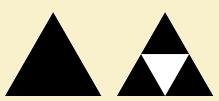If we continue this process again and again, we get more complicated figure, known as Sierpinski triangle.Any triangle has a topological dimension of 2. Intuitively speaking, in Sierpinski triangle, we find more and more triangles, that try to fit inside a larger triangle. However, because of the "hole" in every iteration, we never actually cover the entire area of the outermost triangle. Hence the Sierpinksi triangle has dimension between 1 & 2.
Lets calculate its dimension. We already know that the scaling factor is 2. So we know the value of S. What about N? We created 3 self similar peices during the first Step 1. So N = 3.

Hence the dimension of Sierpinski Triangle is D = Log(3)/Log(2) = 1.58.
We found another object with fractal (Hausdorff) dimension!

Hope you found the idea of "fractal" dimension interesting. We conclude by citing (Hausdorff) dimension of some of the objects you see (and some that you eat!) in daily life.

 Object Hausdorff Dimension Bread2.69 Brocolli2.66 LeavesBetween 1.7 & 1.9 Stock Price Changes for Coca-Cola (Year-2002, Range-200 Days)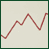0.634

 Quick Comment : * Name Email ( Your email id is safe with us! ) * Comments * Type the number of characters in the word "HUMAN"